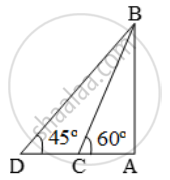# A boat is being rowed away from a cliff 150m high. At the top of the cliff the angle of depression of the boat changes from 60º to 45º in 2 minutes. Find the speed of the boat. - Mathematics

Sum

A boat is being rowed away from a cliff 150m high. At the top of the cliff the angle of depression of the boat changes from 60º to 45º in 2 minutes. Find the speed of the boat.

#### Solution

Let AB be the cliff and C and D be the two positions of the ship. Then, AB = 150 m,

∠ACB = 60º and ∠ADB = 45º.Now,

\frac{AD}{AB}=\text{cot }45^\text{o}=1

\Rightarrow \frac{AD}{150}=1\Rightarrow AD=150 m.

\frac{AC}{AB}=\text{cot 60}^\text{o}=\frac{1}{\sqrt{3}}\Rightarrow\frac{AC}{150}=\frac{1}{\sqrt{3}}

\Rightarrow AC=\frac{150}{\sqrt{3}}=50\sqrt{3}=\text{ }86.6m

∴ CD = AD – AC = (150 – 86.6) m = 63.4 m

Thus, distance covered in 2 min. = 63.4 m

∴ Speed of the boat

=( \frac{63.4}{2}\times \frac{60}{1000} )

Concept: Heights and Distances
Is there an error in this question or solution?